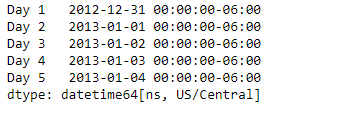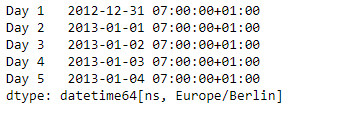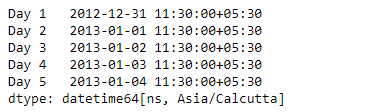# Python | Pandas Series.dt.tz_convert

• Last Updated : 20 Mar, 2019

`Series.dt` can be used to access the values of the series as datetimelike and return several properties. Pandas` Series.dt.tz_convert()` function convert tz-aware Datetime Array/Index from one time zone to another.

Syntax: Series.dt.tz_convert(*args, **kwargs)

Parameter :

tz : Time zone to convert timestamps to.

Returns : same type as self

Example #1: Use `Series.dt.tz_convert()` function to convert the timezone of the timestamps in the given series object.

 `# importing pandas as pd ` `import` `pandas as pd ` ` `  `# Creating the Series ` `sr ``=` `pd.Series(pd.date_range(``'2012-12-31 00:00'``, periods ``=` `5``, freq ``=` `'D'``, ` `                            ``tz ``=` `'US / Central'``)) ` ` `  `# Creating the index ` `idx ``=` `[``'Day 1'``, ``'Day 2'``, ``'Day 3'``, ``'Day 4'``, ``'Day 5'``] ` ` `  `# set the index ` `sr.index ``=` `idx ` ` `  `# Print the series ` `print``(sr) `

Output :Now we will use `Series.dt.tz_convert()` function to convert the timestamps in the given series object to ‘Europe/Berlin’.

 `# convert to 'Europe / Berlin' ` `result ``=` `sr.dt.tz_convert(tz ``=` `'Europe / Berlin'``) ` ` `  `# print the result ` `print``(result) `

Output :As we can see in the output, the `Series.dt.tz_convert()` function has successfully converted the timezone of the timestamps in the given series object to the target timezone.

Example #2 : Use `Series.dt.tz_convert()` function to convert the timezone of the timestamps in the given series object.

 `# importing pandas as pd ` `import` `pandas as pd ` ` `  `# Creating the Series ` `sr ``=` `pd.Series(pd.date_range(``'2012-12-31 00:00'``, periods ``=` `5``, freq ``=` `'D'``, ` `                            ``tz ``=` `'US / Central'``)) ` ` `  `# Creating the index ` `idx ``=` `[``'Day 1'``, ``'Day 2'``, ``'Day 3'``, ``'Day 4'``, ``'Day 5'``] ` ` `  `# set the index ` `sr.index ``=` `idx ` ` `  `# Print the series ` `print``(sr) `

Output :Now we will use `Series.dt.tz_convert()` function to convert the timestamps in the given series object to ‘Asia/Calcutta’.

 `# convert to 'Asia / Calcutta' ` `result ``=` `sr.dt.tz_convert(tz ``=` `'Asia / Calcutta'``) ` ` `  `# print the result ` `print``(result) `

Output :As we can see in the output, the `Series.dt.tz_convert()` function has successfully converted the timezone of the timestamps in the given series object to the target timezone.

My Personal Notes arrow_drop_up
Recommended Articles
Page :